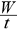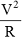# Physics

### Chapter : 1. Electricity

#### Electrical Power

Electrical Power : The rate of loss of energy in an electrical circuit is called electrical power. It is denoted by'P'
p == I2 R = IV =units of power = joule/sec, watt, horse power
1 watt = 1 joule/sec, 1 HP = 746 watt
unit of electrical energy = watt second, kilowatt hour
1 kilowatt hour (kwh) = 3.6 x 106 Joule